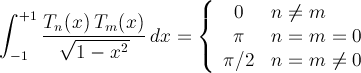﻿OrthogonalPolynomials.ChebyshevT MethodComputes the value of a Cebyshev polynomial.

Namespace:  Meta.Numerics.Functions
Assembly:  Meta.Numerics (in Meta.Numerics.dll) Version: 4.1.4Syntax
```public static double ChebyshevT(
int n,
double x
)```

#### Parameters

n
Type: SystemInt32
The order, which must be non-negative.
x
Type: SystemDouble
The argument, which must lie in the closed interval between -1 and +1.

#### Return Value

Type: Double
The value of Tn(x).Exceptions
ExceptionCondition
ArgumentOutOfRangeExceptionn is negative, or x lies outside [-1,+1].Remarks

Chebyshev polynomials are orthogonal on the interval [-1,1] with the weight (1-x2)-1/2.See Also